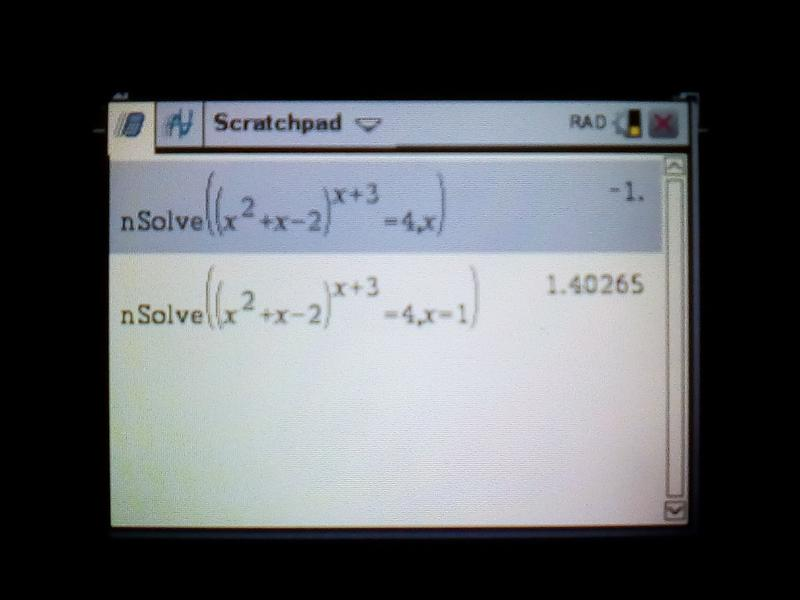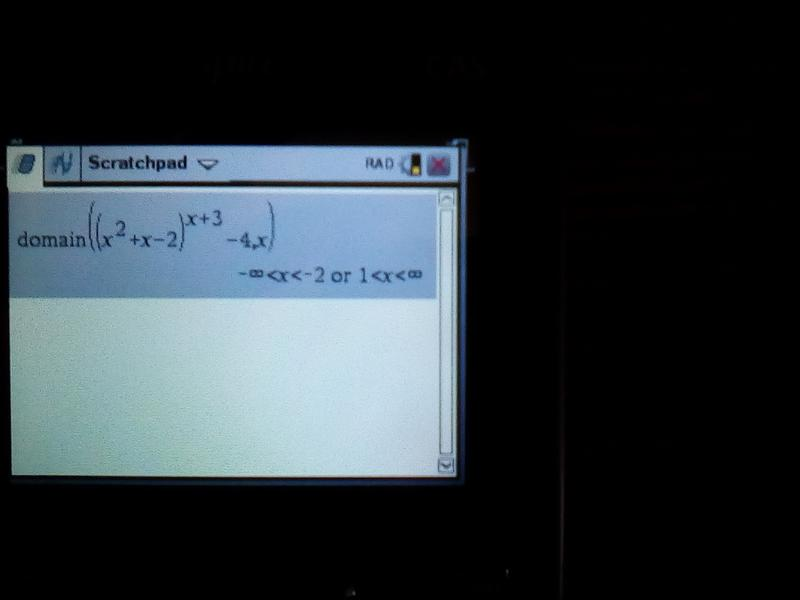[Bug]-Missing root in solving equation
03-01-2019, 10:45 PM (This post was last modified: 03-15-2019 11:35 AM by pvp100288840.)
Post: #1
 pvp100288840Junior Member Posts: 13 Joined: Mar 2019
[Bug]-Missing root in solving equation
As following equation like this:
Quote:(x^2+x-1)^(x+3)=1
The correct answer is:-3,-2,-1,1,but Prime(Xcas) returned[-3 -2 1]
I tried it on different calculator/soft wear (like wolframalpha and CP400),but only a few can solve it correctly(Like nspire CX CAS...)
Almost all calculator/soft wear missing this root:'-1'
Do you guys want to have a try?I havn't test it on 50g XD
03-03-2019, 02:28 AM
Post: #2
 Helge GabertSenior Member Posts: 467 Joined: Dec 2013
RE: [Bug]-Missing root in solving equation
You mean (x^2+x-1)^(x+3)=1, right?

The general solve() routine does indeed miss x=-1 as a valid root - - I suspect this is because the function returns complex results in the interval from around x=-1.618 ... to x=.618 ..., and only at x=-1 does the function disappear. (There is also a real result at x=0).

Of course fsolve() does not miss -1, given a suitable starting value, e.g., if you suspect a root at -1, you can always try

fsolve((x^2+x-1)^(x+3)=1, x=-1.1) returns -1.
03-03-2019, 06:04 PM (This post was last modified: 03-03-2019 06:47 PM by Helge Gabert.)
Post: #3
 Helge GabertSenior Member Posts: 467 Joined: Dec 2013
RE: [Bug]-Missing root in solving equation
P.S. I don't see this as a bug. In fact, it would be a tall order to expect a root-finding algorithm to deliver all roots for any arbitrary real (let alone complex-valued) function.
03-03-2019, 07:25 PM
Post: #4anderinJunior Member Posts: 12 Joined: Dec 2013
RE: [Bug]-Missing root in solving equation
I've tested it on the TI Voyage 200 and it solves it correctly. Curious...
03-04-2019, 05:35 PM
Post: #5
 parisseSenior Member Posts: 1,206 Joined: Dec 2013
RE: [Bug]-Missing root in solving equation
As explained by Helge, the expression is not (real-)defined in the vicinity of -1. You could not find it by bisection for example. And how do you solve this equation? Taking the logarithm of both side, you can understand why there is a problem at x=-1.
03-15-2019, 11:35 AM
Post: #6
 pvp100288840Junior Member Posts: 13 Joined: Mar 2019
RE: [Bug]-Missing root in solving equation
(03-03-2019 07:25 PM)anderin Wrote:  I've tested it on the TI Voyage 200 and it solves it correctly. Curious...

In fact,all TI calculator ,including v200 && nspire ,they all solved it correctly.But HP's and CASIO's not
03-15-2019, 11:41 AM
Post: #7
 pvp100288840Junior Member Posts: 13 Joined: Mar 2019
RE: [Bug]-Missing root in solving equation
(03-03-2019 02:28 AM)Helge Gabert Wrote:  You mean (x^2+x-1)^(x+3)=1, right?

The general solve() routine does indeed miss x=-1 as a valid root - - I suspect this is because the function returns complex results in the interval from around x=-1.618 ... to x=.618 ..., and only at x=-1 does the function disappear. (There is also a real result at x=0).

Of course fsolve() does not miss -1, given a suitable starting value, e.g., if you suspect a root at -1, you can always try

fsolve((x^2+x-1)^(x+3)=1, x=-1.1) returns -1.

Thanks a lot! But all TI calculators can solve this correctly(like TI-89).I wonder if it means that HP's(and also CASIO's) CAS algorithm do worse than TI?
Besides,How can I compare the CAS algorithm?
03-17-2019, 02:31 AM
Post: #8
 Helge GabertSenior Member Posts: 467 Joined: Dec 2013
RE: [Bug]-Missing root in solving equation
Yes, that is nice that the TI family of calculators can apparently solve this equation for all roots on its domain!

However, I am not aware of any algorithm which returns all roots (globally, and guaranteed!) on the entire domain of any arbitrary real function (not just a polynomial), which may also be complex-valued. If such an algorithm existed, and if it could be easily implemented, and if it wouldn't be too slow, I'm sure the HP Prime CAS system would have it incorporated.

I don't own any TIs, so I can't give you a counter example, but I'm sure there is a function or two where "even" a TI calc will have to pass, i.e., not finding all global roots. Maybe somebody can come up with one . . .
03-18-2019, 01:07 PM
Post: #9
 roadrunnerSenior Member Posts: 398 Joined: Jun 2015
RE: [Bug]-Missing root in solving equation
(03-17-2019 02:31 AM)Helge Gabert Wrote:  Maybe somebody can come up with one . . .

(x^2+x-2)^(x+3)) = 4 has a solution at x=-1, but:03-18-2019, 04:01 PM (This post was last modified: 03-18-2019 04:15 PM by Helge Gabert.)
Post: #10
 Helge GabertSenior Member Posts: 467 Joined: Dec 2013
RE: [Bug]-Missing root in solving equation
Excellent!

The Prime does find x=1.402 . . . (general solve) and x=-1 (fsolve or solve with a single starting value of x=-3, for example), and also certain complex solutions, e.g., with starting values of x=-1.5, or x=-7.
03-18-2019, 05:13 PM (This post was last modified: 05-22-2022 02:21 PM by Dave Hicks.)
Post: #11
 AriesMember Posts: 158 Joined: Oct 2014
RE: [Bug]-Missing root in solving equationWith SOLVE there is an underflow (ignored and zero substituted), better using numerical solver in such a caseBest,

Aries03-18-2019, 07:48 PM
Post: #12
 Helge GabertSenior Member Posts: 467 Joined: Dec 2013
RE: [Bug]-Missing root in solving equation
Good to know! But this is not different from using fsolve() with the Prime, and a single starting value.

In the example given by roadrunner, the TI doesn't appear to deliver all roots over the reals in one fell swoop.
03-19-2019, 02:33 PM
Post: #13
 AriesMember Posts: 158 Joined: Oct 2014
RE: [Bug]-Missing root in solving equation
-1 is not part of the (Reals) domain:After factoring:With no guesses !
Best,

Aries03-19-2019, 03:46 PM
Post: #14
 Helge GabertSenior Member Posts: 467 Joined: Dec 2013
RE: [Bug]-Missing root in solving equation
?

I still don't see the Ti delivering both real roots [-1., 1.402...] with one command (only separately, with manipulation, just like the Prime).

By the way, for this example,

gets both real roots.
03-20-2019, 06:22 AM
Post: #15
 AriesMember Posts: 158 Joined: Oct 2014
RE: [Bug]-Missing root in solving equation
The (variable) base cannot be negative, it's to be posed >0 hence -1 is not part of the domaine^((x+3)*ln(x^2+x-2)), for x=-1 you get e^(2*ln(-2)) where ln(-2)=ln(2)+pi*i (i is the imaginary unit)03-20-2019, 07:57 AM
Post: #16ijabbottSenior Member Posts: 1,113 Joined: Jul 2015
RE: [Bug]-Missing root in solving equation
(03-20-2019 06:22 AM)Aries Wrote:  The (variable) base cannot be negative, it's to be posed >0 hence -1 is not part of the domaine^((x+3)*ln(x^2+x-2)), for x=-1 you get e^(2*ln(-2)) where ln(-2)=ln(2)+pi*i (i is the imaginary unit)So how do you explain the TI's ability to include -1 amongst the solutions of (x^2+x-1)^(x+3)=1?

— Ian Abbott
03-20-2019, 03:07 PM
Post: #17
 Helge GabertSenior Member Posts: 467 Joined: Dec 2013
RE: [Bug]-Missing root in solving equation
Good point regarding -1 as a root of the original function!

Also, as shown in a previous post by Aries for the second function, The Ti returns -1 as a root with nSolve().

These functions have a range which is complex-valued for a part of their domain (if you accept the domain to include all of R). Sometimes, the (generally) complex solution resolves to a real number, depending on the occurrence of whole powers of i or -1, or a combination of \pi, or e in the context of Euler's identity. This happens for the second function at x=-1, and also at x=0 (although zero is, of course, not a root).

If you restrict the domain to not include any complex results in the range - - well, then you can ignore any real roots in that interval.
03-29-2019, 04:17 PM
Post: #18
 AriesMember Posts: 158 Joined: Oct 2014
RE: [Bug]-Missing root in solving equation
What I generally do (if the equation is particularly "unpleasant") is plot the func and try to estimate a zero using two initial values, most of the time algorithms converge to the nearest solution.
As an indication, whether the equation is complex and has only one unknown, better use nsolve instead of solve.
Nsolve looks for just ONE solution numerically and in a number of cases a solution can't be found.
Nsolve takes several numerical algorithms (Newton, bisection, fixed-point, and so on), in many cases they converge smartly, in others they fail to converge.
It's a big help to provide an initial guess, a good value can "speed up" calculations and help the algorithm to converge to a solution.
A further point is that different (numerical) algorithms get different error margins; if a result is displayed using 14 digits, that does not mean we get a precision of the magnitude of 10^(-14).
I guess the value should be around 10^(-5) or 10^(-6) from the exact result.
Best,

Aries« Next Oldest | Next Newest »

User(s) browsing this thread: 1 Guest(s)